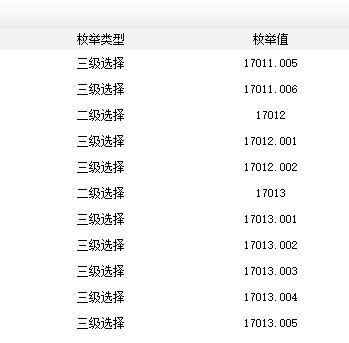dedecms织梦自定义表单添加地区三级联动正确的修复方案

# dedecms织梦自定义表单添加地区三级联动正确的修复方案

2、修改的文件：dede/templets/diy_field_edit.htm 大概在127行，很明显已经注释掉，我们把注释去掉，然后放到“多选框”下面。

 1 2 3 4 5 6 else if(\$fielddata=='addon')              {                \$fields[\$field] = " 其它附件";              }

在其 下面 添加如下代码

 1 2 3 else if(\$fielddata=='stepselect')       {          \$fields[\$field] = \$em_nativeplaces[GetEnumsTypes(\$fields[\$field])['top']].\$em_nativeplaces[GetEnumsTypes(\$fields[\$field])['son']].\$em_nativeplaces[GetEnumsTypes(\$fields[\$field])['sun']];        }

打开 dede/diy_list.php文件，找到

else if (\$action == 'list')
{

include_once DEDEINC.'/datalistcp.class.php';
在下面添加如下代码

include_once(DEDEDATA.'/enums/nativeplace.php');
include_once(DEDEINC."/enums.func.php");

/**
*  获取联动表单两级数据的父类与子类
*
* @access    public
* @param     string  \$v
* @return    array
*/
function GetEnumsTypes(\$v)
{
\$rearr['top'] = \$rearr['son'] = \$rearr['sun'] = 0;
if(\$v==0) return \$rearr;
if(\$v%500==0) {
\$rearr['top'] = \$v;
}
else{

\$rearr['son'] = \$v;
\$rearr['top'] = \$v – (\$v%500);
}
return \$rearr;
}

/**
*  获取联动表单两级数据的父类与子类
*
* @access    public
* @param     string  \$v
* @return    array
*/
function GetEnumsTypes(\$v)
{
\$rearr['top'] = \$rearr['son'] = \$rearr['sun'] = 0;
if(\$v==0) return \$rearr;
if(\$v%500==0) {
\$rearr['top'] = \$v;
}
else{
\$rearr['sun'] = \$v;
\$rearr['son'] = intval(\$v);
\$rearr['top'] = \$v – (\$v%500);
}
return \$rearr;
}# 微信扫码购买修复包